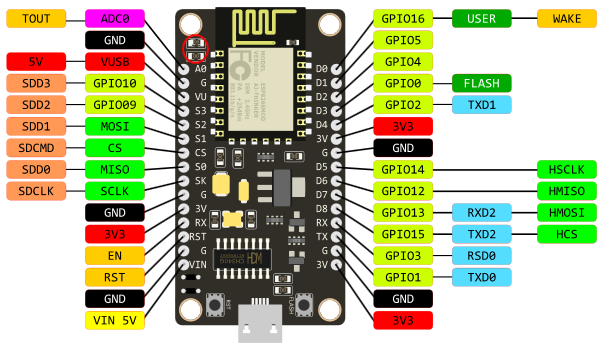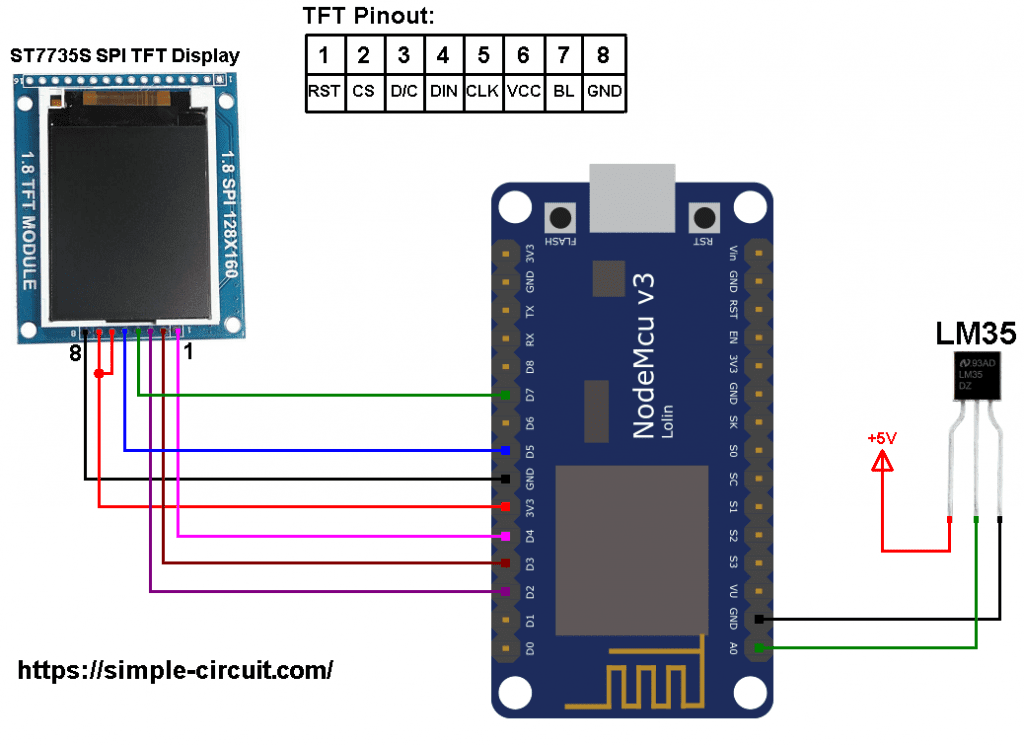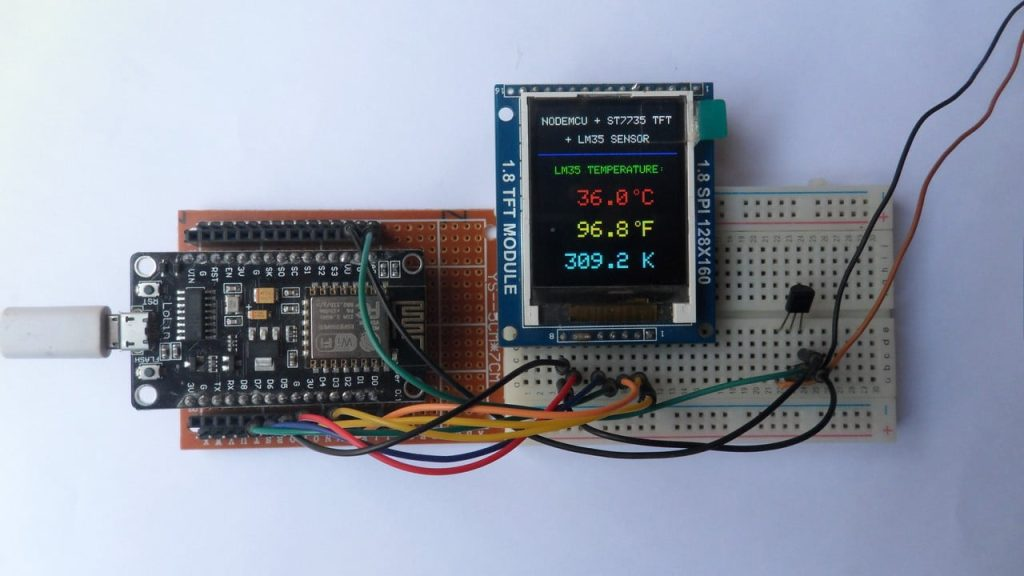# NodeMCU with ST7735 TFT and LM35 Analog Temperature Sensor

This NodeMCU project shows how to build a simple thermometer using LM35 analog temperature sensor where temperature value is printed on ST7735 TFT display (in degree Celsius, Kelvin and degree Fahrenheit).
The ST7735 TFT is a color display with resolution of 128×160 pixel, it communicates with the master device using SPI protocol.

TFT: Thin-Film Transistor
SPI: Serial Peripheral Interface

To see how to interface the NodeMCU board with the ST7735 TFT display, visit this post:
Interfacing ESP8266 NodeMCU with ST7735 TFT

The LM35 temperature sensor is a three pin device (VCC, OUT and GND) with an output voltage linearly related to Centigrade temperature. Since the LM35 output varies with dependent to the temperature, we need an ADC (Analog-to-Digital Converter) module to measure this voltage. The NodeMCU microcontroller (ESP8266EX) has one ADC module with 10-bit resolution.

The LM35 output has linear +10mV/°C scale factor means the following:
If the output voltage =   10mV —> temperature =   1°C
If the output voltage = 100mV —> temperature = 10°C
If the output voltage = 200mV —> temperature = 20°C
If the output voltage = 370mV —> temperature = 37°C
and so on.

LM35 Futures (from datasheet):

• Calibrated Directly in ° Celsius (Centigrade)
• Linear + 10 mV/°C Scale Factor
• 0.5°C Ensured Accuracy (at +25°C)
• Rated for Full −55°C to +150°C Range
• Suitable for Remote Applications
• Low Cost Due to Wafer-Level Trimming
• Operates from 4 to 30 V
• Less than 60-μA Current Drain
• Low Self-Heating, 0.08°C in Still Air
• Nonlinearity Only ±¼°C Typical
• Low Impedance Output, 0.1 Ω for 1 mA Load

The ADC module converts analog data into digital data. The ESP8266EX MCU has a 10-bit ADC module with one analog channel. Negative and positive references of the ADC module are 0V (GND) and 1.0V respectively. That means a 0V is represented by 0 and 1.0V (or higher but we shouldn’t apply more than that) is represented by 1024.

LoLin version of the NodeMCU board analog input is A0 (or ADC0), it’s connected to the ESP8266EX microcontroller through voltage divider of 2 resistors (220k and 100k ohm) as shown in the image below (red circle).
With the built-in voltage divider, the NodeMCU analog channel supports inputs from 0 to 3.3V.Also, using the LoLin NodeMCU we can supply the LM35 sensor with 5V that comes from pin 5V (or VUSB).

Hardware Required:

• ESP8266 NodeMCU board
• ST7735 TFT display module
• LM35 temperature sensor   —->   datasheet
• micro USB cable (for programming and powering the circuit)
• 5V source (optional)
• Jumper wires

NodeMCU with ST7735 TFT and LM35 sensor circuit:
Project circuit schematic diagram is shown below.

The LM35 sensor has 3 pins (from left to right):
Pin 1 is power supply pin, connected to external 5V power source positive terminal (don’t forget to connect its negative terminal to any of the NodeMCU ground pins).
In the absence of 5V source, this pin may be connected to NodeMCU 3V3 pin (not recommended because LM35 sensor voltage range is from 4V to 36V).
Pin 2: output pin, connected to NodeMCU pin A0 (ADC0),
Pin 3: GND (ground), connected to NodeMCU GND pin.The ST7735S shown in project circuit diagram has 8 pins: (from right to left): RST (reset), CE (chip enable), DC (or D/C: data/command), DIN (data in), CLK (clock), VCC, BL (back light) and Gnd (ground).

The ST7735 display is connected to the NodeMCU board as follows:
RST pin is connected to D4 (ESP8266EX GPIO2),
CS pin is connected to D3 (ESP8266EX GPIO0),
D/C pin is connected to D2 (ESP8266EX GPIO4),
DIN (MOSI) pin is connected to D7 (ESP8266EX GPIO13),
CLK (SCK) pin is connected to D5 (ESP8266EX GPIO14),
VCC and BL are connected to pin 3V3,
GND is connected to pin GND of the NodeMCU board.

Pins D5 (GPIO14) and D7 (GPIO13) are hardware SPI module pins of the ESP8266EX microcontroller respectively for SCK (serial clock) and MOSI (master-out slave-in).

NodeMCU with ST7735 TFT and LM35 sensor code:
The following Arduino code requires two libraries from Adafruit Industries:
The first library is a driver for the ST7735 TFT display which can be installed from Arduino IDE library manager (Sketch —> Include Library —> Manage Libraries …, in the search box write “st7735” and install the one from Adafruit).

The second library is Adafruit graphics library which can be installed also from Arduino IDE library manager.

The same thing for the other library file.

Hints:
The previous 2 libraries are included in the main code as follows:

The connection of ST7735 TFT display with the NodeMCU is as shown below where the display is connected to hardware SPI module of the NodeMCU (pins: SCK and MOSI):

Reading analog voltage using ADC module gives a number between 0 and 1023 (10-bit resolution), 0V is represented by 0 and 3.3V is represented by 1023. Converting back the ADC digital value is easy, we can use the following equation:

This voltage (in milliVolts) represents the temperature value in tenths degree Celsius (output value of “273” equals 27.3 °Celsius).

The temperature in tenths Kelvin = (tenth °Celsius) + 2732 (because: K = °C + 273.16).

To get the actual value of each quantity we’ve to divide it by 10. The line below shows an example for temperature in Kelvin:

We get the first 3 digits by dividing the tenths value by 10, and the tenths number (number after the decimal point) of the actual temperature value is equal to the reminder of that division (tenths value % 10).

The resolution of this thermometer is about 0.3°C.

Full Arduino Code:This site uses Akismet to reduce spam. Learn how your comment data is processed.